# 定义和距离公式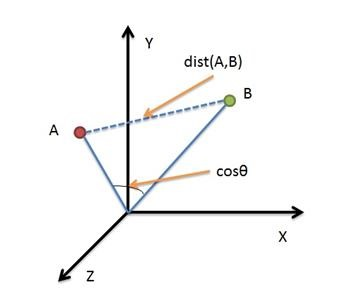• 二维平面上点a(x1,y1)与b(x2,y2)间的欧氏距离：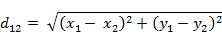• 三维空间点a(x1,y1,z1)与b(x2,y2,z2)间的欧氏距离：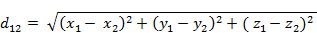• n维空间点a(x11,x12,…,x1n)与b(x21,x22,…,x2n)间的欧氏距离（两个n维向量）：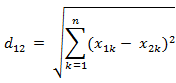## Python计算欧式距离

import numpy
v1=[1 1]
v2=[2,2]
vec1=numpy.array(v1)
vec2=numpy.array(v2)
ist = numpy.linalg.norm(vec1 - vec2)

>>> ist
1.4142135623730951

# 2. 曼哈顿距离（Manhattan distance）

## 描述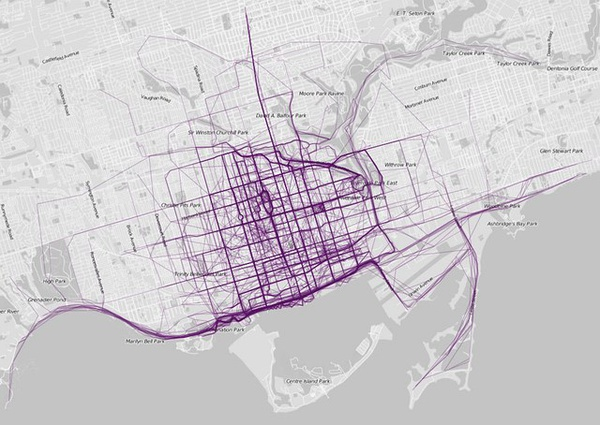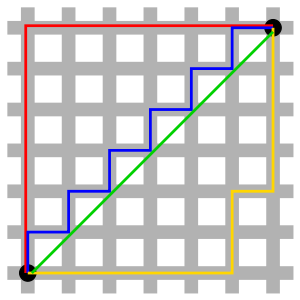## 定义和距离公式

• 二维平面两点a(x1,y1)与b(x2,y2)间的曼哈顿距离：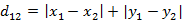• n维空间点a(x11,x12,…,x1n)与b(x21,x22,…,x2n)的曼哈顿距离：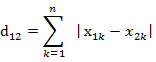# 3. 切比雪夫距离（Chebyshev distance）

## 描述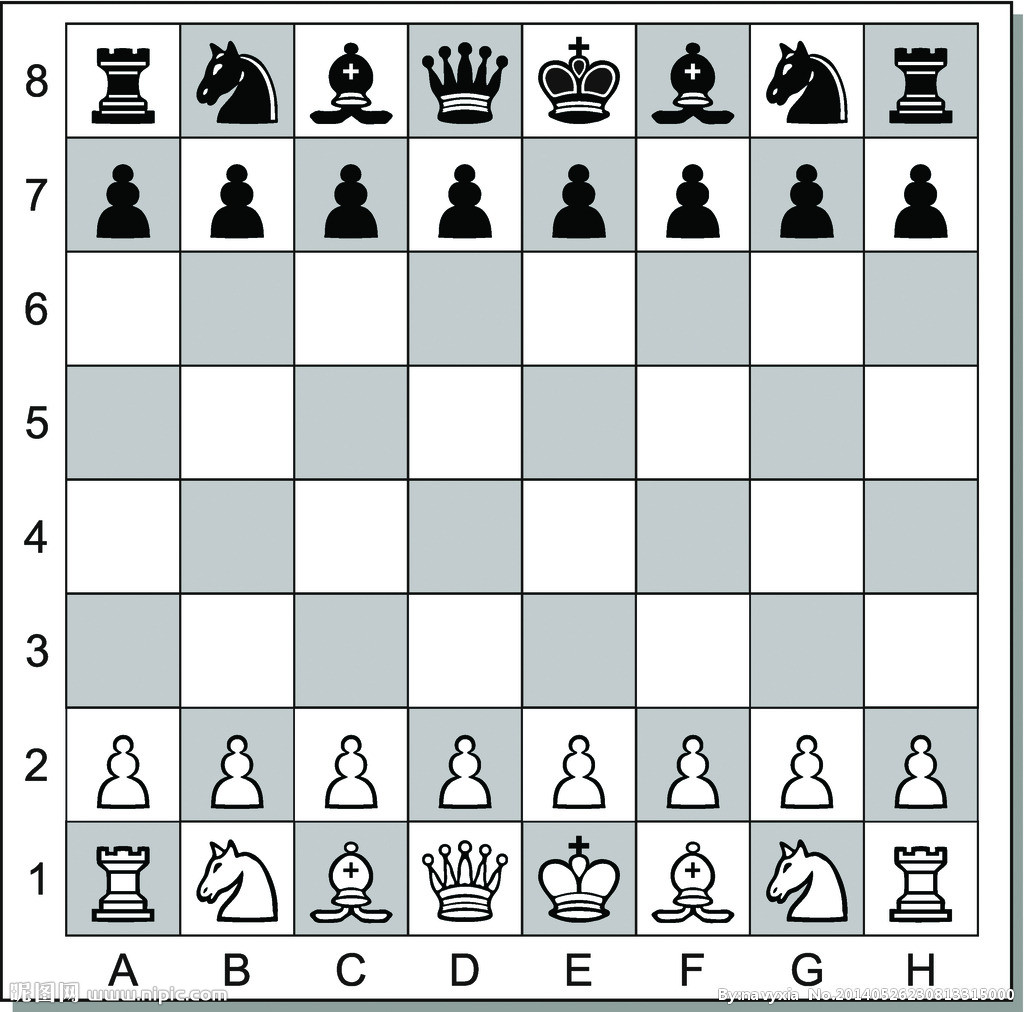## 定义和距离公式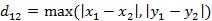n维空间点a(x11,x12,…,x1n)与b(x21,x22,…,x2n)的切比雪夫距离：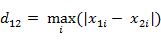# 4. 闵可夫斯基距离（Minkowski Distance）

## 描述

The Minkowski distance is a metric on Euclidean space which can be considered as a generalization of both the Euclidean distance and the Manhattan distance.

The Minkowski distance can also be viewed as a multiple of the power mean of the component-wise differences between P and Q.

## 定义和距离公式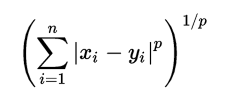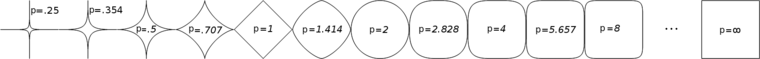• p=1时，闵式距离即为曼哈顿距离；
• p=2时，闵式距离即为欧氏距离；
• p=∞时，闵式距离即为切比雪夫距离；

## 缺点

1. 将各个分量的量纲(scale)，也就是“单位”当作相同的看待了。
2. 没有考虑各个分量的分布（期望，方差等)可能是不同的。

# 5. 标准化欧氏距离（Standardized Euclidean distance）

## 定义和距离公式

X* = (X-m)/s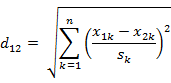# 6. 马氏距离/马哈拉诺比斯距离（Mahalanobis Distance）

## 描述

The Mahalanobis distance is a descriptive statistic that provides a relative measure of a data point’s distance (residual) from a common point.

## 图的认识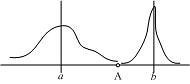## 定义和距离公式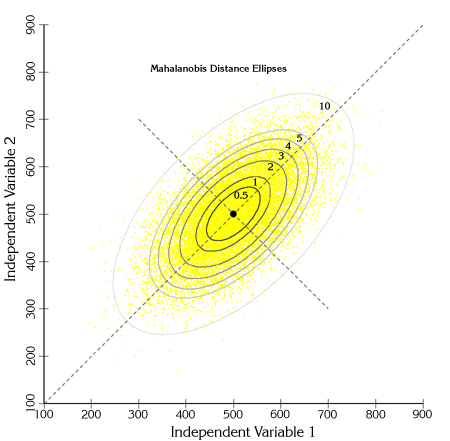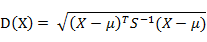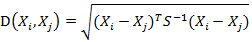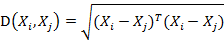## 欧式距离&马氏距离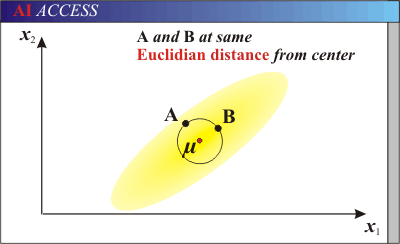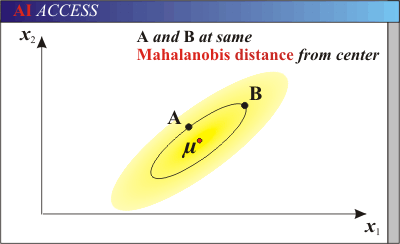## 马氏距离特点

1. 量纲无关，排除变量之间的相关性的干扰
2. 马氏距离的计算是建立在总体样本的基础上的，如果拿同样的两个样本，放入两个不同的总体中，最后计算得出的两个样本间的马氏距离通常是不相同的，除非这两个总体的协方差矩阵碰巧相同；
3. 计算马氏距离过程中，要求总体样本数大于样本的维数，否则得到的总体样本协方差矩阵逆矩阵不存在，这种情况下，用欧式距离计算即可。

# 7.汉明距离（Hamming distance）

## 描述和定义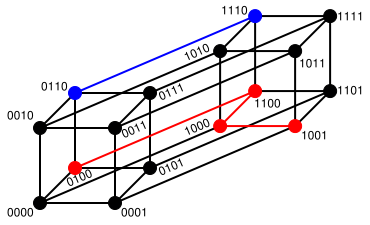The Hamming distance between “toned” and “roses” is 3.

# 8. 夹角余弦（Cosine）

## 定义和公式

• 在二维空间中向量A(x1,y1)与向量B(x2,y2)的夹角余弦公式：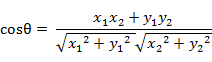• 扩展到n维，则两个n维样本点a(x11,x12,…,x1n)和b(x21,x22,…,x2n)的夹角余弦为：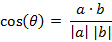即：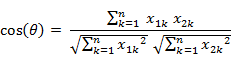# 9.相关系数 ( Correlation coefficient )

## 描述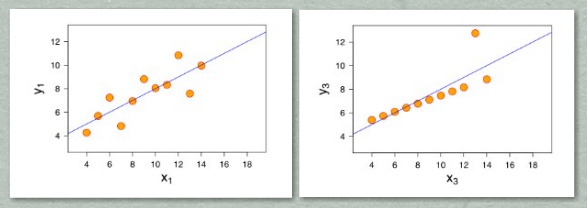## 定义和公式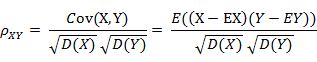## Python算法

from numpy import *
a = array([1,2,3,4,6,7,8,9])
b = array([2,4,6,8,10,12,13,15])
c = array([-1,-2,-2,-3,-4,-6,-7,-8])
>>> corrcoef([a,b,c])
array([[ 1.        ,  0.99535001, -0.9805214 ],
[ 0.99535001,  1.        , -0.97172394],
[-0.9805214 , -0.97172394,  1.        ]])

# 10. 杰卡德相似系数(Jaccard similarity coefficient)和杰卡德距离(Jaccard distance)

## 定义和公式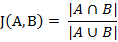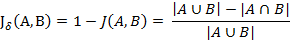## 应用

M11：样本A与B都是1的维度的个数
M01：样本A是0，样本B是1的维度的个数
M10：样本A是1，样本B是0 的维度的个数
M00：样本A与B都是0的维度的个数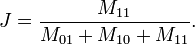# 11. 皮尔逊系数(Pearson Correlation Coefficient)

0.8-1.0 极强相关
0.6-0.8 强相关
0.4-0.6 中等程度相关
0.2-0.4 弱相关
0.0-0.2 极弱相关或无相关

## 定义和公式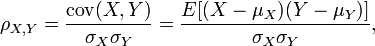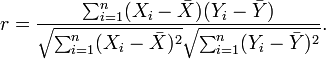## Python代码实现

from math import sqrt

def multipl(a,b):
sumofab=0.0
for i in range(len(a)):
temp=a[i]*b[i]
sumofab+=temp
return sumofab

def corrcoef(x,y):
n=len(x)
#求和
sum1=sum(x)
sum2=sum(y)
#求乘积之和
sumofxy=multipl(x,y)
#求平方和
sumofx2 = sum([pow(i,2) for i in x])
sumofy2 = sum([pow(j,2) for j in y])
num=sumofxy-(float(sum1)*float(sum2)/n)
#计算皮尔逊相关系数
den=sqrt((sumofx2-float(sum1**2)/n)*(sumofy2-float(sum2**2)/n))
return num/den

x = [0,1,0,3]
y = [0,1,1,1]

print corrcoef(x,y) #0.471404520791

# 12. 信息熵(Information Entropy)

## 定义和公式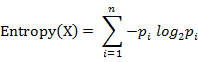# 总结

空间：欧氏距离;

## 思维导图：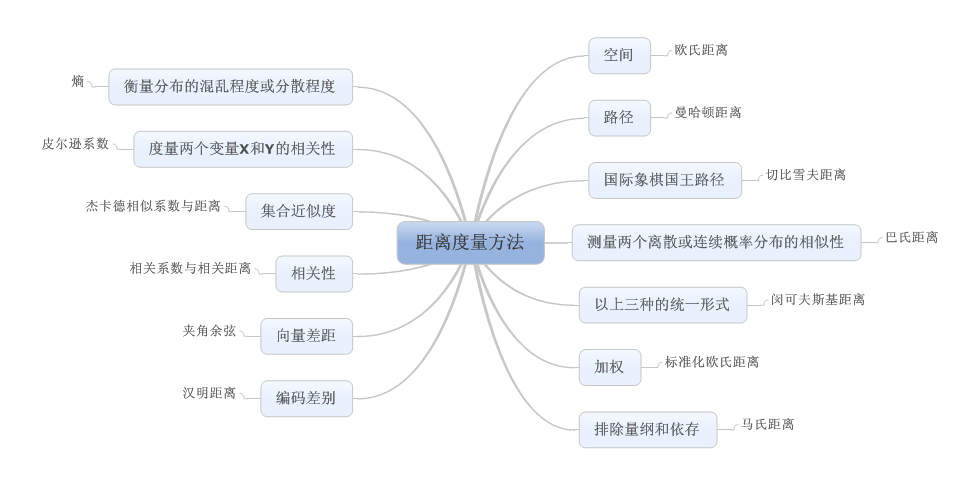## 致谢

• http://www.manyjar.com:8080/search/mahout-distribution-0.9-src.zip.html‘>mahout源码
• http://blog.csdn.net/v_july_v/article/details/8203674/‘>从K近邻算法、距离度量谈到KD树、SIFT+BBF算法
• http://arthur503.github.io/blog/2013/10/03/Statistical-Methods-appendix-hilbert-space-and-more-spaces.html‘>统计学习方法BR-附录：希尔伯特空间、欧几里得空间和巴拿赫空间【存疑】
• a href=’http://zh.wikipedia.org/wiki/%E6%9B%BC%E5%93%88%E9%A0%93%E8%B7%9D%E9%9B%A2‘>曼哈顿距离
• http://zh.wikipedia.org/wiki/%E5%88%87%E6%AF%94%E9%9B%AA%E5%A4%AB%E8%B7%9D%E7%A6%BB‘>切比雪夫距离
• http://en.wikipedia.org/wiki/List_of_mathematical_symbols‘>List of mathematical symbols
• http://en.wikipedia.org/wiki/Minkowski_distance‘>Minkowski distance
• http://en.wikipedia.org/wiki/Mahalanobis_distance‘>Mahalanobis distance
• http://arthur503.github.io/blog/2013/09/05/edit-distance-how-faraway-are-we-1.html‘>编辑距离：我和你到底有多远？（一）
• 还能输入1000个字符没有更多推荐了，返回首页

©️2019 CSDN 皮肤主题: 编程工作室 设计师: CSDN官方博客扫一扫，手机浏览